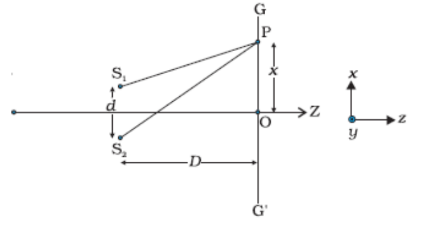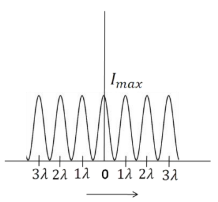# NEET Physics Wave Optics Questions Solved

Watch Physics > Wave Optics VideosBOARD

(i) In Young's double slit exoeriment, deduce the condition for (a) constructive,

and (b) destructive interference at a point on the screen. Draw a graph

showing variation of intensity in the interference pattern against position

'x' on the screen.

(ii) Compare the interference pattern observed in Young's double slit experiment

with single slit diffraction pattern, pointing out three distinguishing features.

(5 marks)

(i)(1/2 mark)

From Figure

Path difference = $\left({\mathrm{S}}_{2}\mathrm{P}-{\mathrm{S}}_{1}\mathrm{P}\right)$

(1/2 mark)

For x,d <<D

${\mathrm{S}}_{2}\mathrm{P}+{\mathrm{S}}_{1}\mathrm{P}=2\mathrm{D}$

so, ${\mathrm{S}}_{2}\mathrm{P}-{\mathrm{S}}_{1}\mathrm{P}=\frac{2\mathrm{xd}}{2\mathrm{D}}=\frac{\mathrm{xd}}{\mathrm{D}}$                                 (1/2 mark)

For constructive interference

$⇒\frac{\mathrm{xd}}{\mathrm{D}}=\mathrm{n\lambda }\phantom{\rule{0ex}{0ex}}⇒\mathrm{x}=\frac{\mathrm{n\lambda D}}{\mathrm{d}}$                                                  (1/2 mark)

For destructive interference ${\mathrm{S}}_{2}\mathrm{P}-{\mathrm{S}}_{1}\mathrm{P}=\left(2\mathrm{n}+1\right)\frac{\mathrm{\lambda }}{2}$

n=0, 1, 2....

(1/2 mark)Path Difference                             (1 mark)

(ii)

(a) The Interference pattern has number of equally spaced bright and

dark bands, while in the diffraction pattern the width of the central

maximum is twice the width of other maxima.                           (1/2 mark)

(b) In Interference all bright fringes are of equal intensity, whereas

in the difraction pattern the intensity falls as order if maxima

increases.                                                                     (1/2 mark)

(c) In Interference pattern, maxima occurs at an angle $\frac{\mathrm{\lambda }}{\mathrm{a}}$,where

a is the slit width, whereas in diffraction pattern, at the same

angle, first minimum occurs. (Here 'a' is the size of the slit.)           (1/2 mark)

`

Difficulty Level:

Crack NEET with Online Course - Free Trial (Offer Valid Till September 22, 2019)